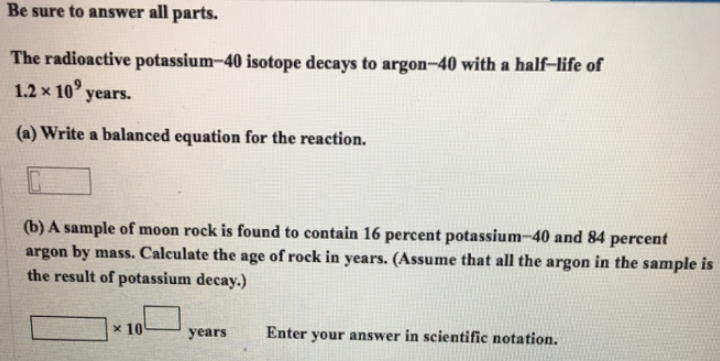# Problem: Be sure to answer all parts.The radioactive potassium-40 isotope decays to argon-40 with a half-life of 1.2 x 109 years. (a) Write a balanced equation for the reaction. (b) A sample of moon rock is found to contain 16 percent potassium-40 and 84 percent argon by mass. Calculate the age of rock in years. (Assume that all the argon in the sample is the result of potassium decay.) Enter your answer in scientific notation.

###### FREE Expert Solution
97% (133 ratings)###### Problem Details

Be sure to answer all parts.

The radioactive potassium-40 isotope decays to argon-40 with a half-life of 1.2 x 109 years.

(a) Write a balanced equation for the reaction.

(b) A sample of moon rock is found to contain 16 percent potassium-40 and 84 percent argon by mass. Calculate the age of rock in years. (Assume that all the argon in the sample is the result of potassium decay.)